IIT JAM Physics MCQ Test 12

# IIT JAM Physics MCQ Test 12

Test Description

## 30 Questions MCQ Test Mock Test Series for IIT JAM Physics | IIT JAM Physics MCQ Test 12

IIT JAM Physics MCQ Test 12 for Physics 2023 is part of Mock Test Series for IIT JAM Physics preparation. The IIT JAM Physics MCQ Test 12 questions and answers have been prepared according to the Physics exam syllabus.The IIT JAM Physics MCQ Test 12 MCQs are made for Physics 2023 Exam. Find important definitions, questions, notes, meanings, examples, exercises, MCQs and online tests for IIT JAM Physics MCQ Test 12 below.
Solutions of IIT JAM Physics MCQ Test 12 questions in English are available as part of our Mock Test Series for IIT JAM Physics for Physics & IIT JAM Physics MCQ Test 12 solutions in Hindi for Mock Test Series for IIT JAM Physics course. Download more important topics, notes, lectures and mock test series for Physics Exam by signing up for free. Attempt IIT JAM Physics MCQ Test 12 | 30 questions in 90 minutes | Mock test for Physics preparation | Free important questions MCQ to study Mock Test Series for IIT JAM Physics for Physics Exam | Download free PDF with solutions
 1 Crore+ students have signed up on EduRev. Have you?
IIT JAM Physics MCQ Test 12 - Question 1

### If α = 0.98. ICO = 6 μA and lR = 100μA for a transistor, then the value of lc will be

Detailed Solution for IIT JAM Physics MCQ Test 12 - Question 1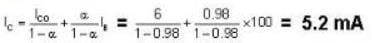IIT JAM Physics MCQ Test 12 - Question 2

### For the given input, the output waveform across the diode shown in the figure. will be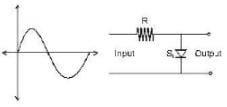Detailed Solution for IIT JAM Physics MCQ Test 12 - Question 2

The diode will not conduct in the negative half of the wave and while it conducts, there will be a small voltage drop across, it shown as 0.06 V on option (D)

IIT JAM Physics MCQ Test 12 - Question 3

### Which of the following option correctly represents the dependence of mobility with temperature?

Detailed Solution for IIT JAM Physics MCQ Test 12 - Question 3

Mobility μh & μp have a temp. dependence & is approximately expressed as μ ∝ T-3/2. Opyion (D) is the correct option.

IIT JAM Physics MCQ Test 12 - Question 4

The graph of [logρ vs. 1/T] can be expressed as:-

Detailed Solution for IIT JAM Physics MCQ Test 12 - Question 4

As (ρ) resistivity = (constant).exp (+Eg/2KT)
Log ρ = log (constant) + Eg/2KT

IIT JAM Physics MCQ Test 12 - Question 5

Which is correct logic diagram of the simplified form of given boolean expression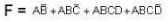Detailed Solution for IIT JAM Physics MCQ Test 12 - Question 5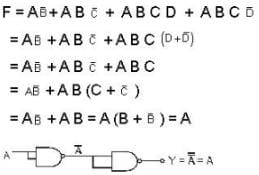IIT JAM Physics MCQ Test 12 - Question 6

F(ABC) = (A + B + AB) (A + C + AC) has simplified expression

Detailed Solution for IIT JAM Physics MCQ Test 12 - Question 6

F(A, BC) = [A + B(1 +A)] [A +C (1+A)]
=  (A+B) (A+C) = A + AB + BC + AC
= A(A+B) + BC+AC
= A + AC + BC
= A(1+C) + AC
= A + BC

IIT JAM Physics MCQ Test 12 - Question 7

An OPAMP has a slew rate of 5 V/μs. The largest sinewave output voltage possible at a frequency of 1 MHz is

Detailed Solution for IIT JAM Physics MCQ Test 12 - Question 7

V = Vm sin ωt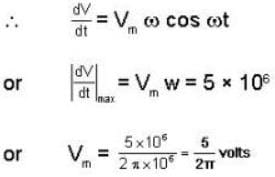IIT JAM Physics MCQ Test 12 - Question 8

For the logic circuit shown in the figure below, the output Y is equal to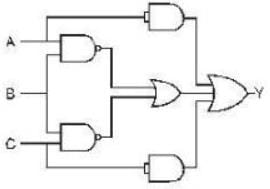Detailed Solution for IIT JAM Physics MCQ Test 12 - Question 8IIT JAM Physics MCQ Test 12 - Question 9

The output voltage of an OPAMP for input voltage of Vi1 = 150 μV , Vi2 = 140μV if the amplifier has a differential gain of Ad = 4000 and the value of CMRR is 100, is

Detailed Solution for IIT JAM Physics MCQ Test 12 - Question 9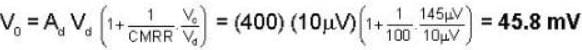IIT JAM Physics MCQ Test 12 - Question 10

A non-inverting op-amp summer is shown in the figure. The output voltage Vo will be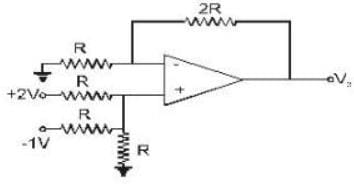Detailed Solution for IIT JAM Physics MCQ Test 12 - Question 10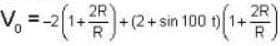= - 6 + 6 + 3 sin 100t = 3 sin 100 t

IIT JAM Physics MCQ Test 12 - Question 11

The Q point of the transistor circuit given below is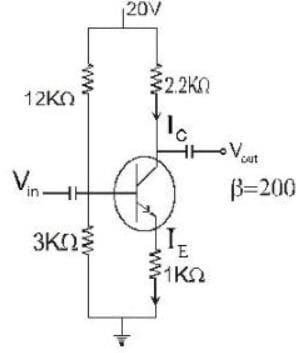Detailed Solution for IIT JAM Physics MCQ Test 12 - Question 11

Using approximation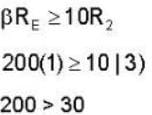So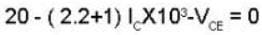...(i)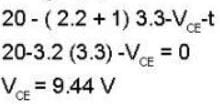IIT JAM Physics MCQ Test 12 - Question 12

The saturation current for the given network is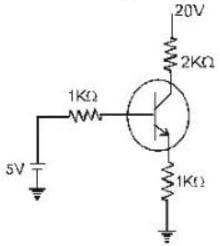Detailed Solution for IIT JAM Physics MCQ Test 12 - Question 12

(Ic) saturation =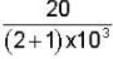20/3 mA= 6.67mA

IIT JAM Physics MCQ Test 12 - Question 13

The value of VCE for the voltage - divider bias configuration is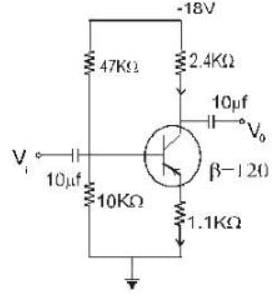Detailed Solution for IIT JAM Physics MCQ Test 12 - Question 13

Testing the condition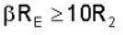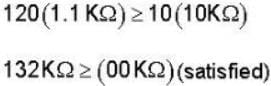Solving for VB we have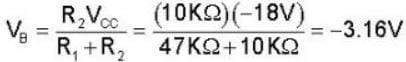Apply KVL around base emitter loop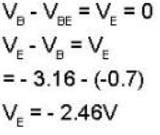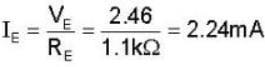For collector - emitter loop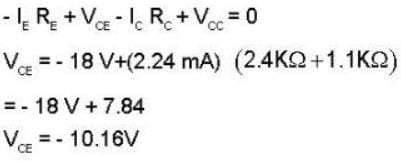IIT JAM Physics MCQ Test 12 - Question 14

For the CB circuit shown below in figure ; The value of ‘ Ic ’ will be:-  ( Assume The transistor to be of silicon)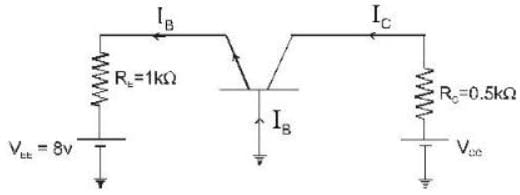Detailed Solution for IIT JAM Physics MCQ Test 12 - Question 14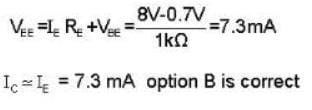IIT JAM Physics MCQ Test 12 - Question 15

For the circuit given below; calculate the value of VCB (Assuming the transistor to be of silicon.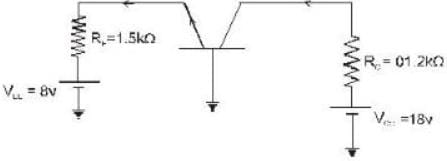Detailed Solution for IIT JAM Physics MCQ Test 12 - Question 15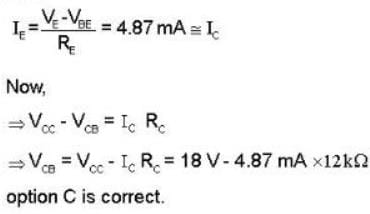*Multiple options can be correct
IIT JAM Physics MCQ Test 12 - Question 16

Choose the correct statement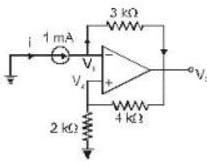Detailed Solution for IIT JAM Physics MCQ Test 12 - Question 16

We note that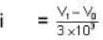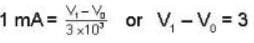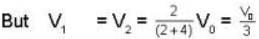so putting in eq. (1), we get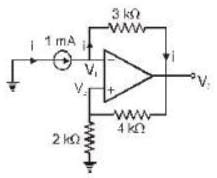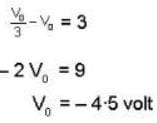and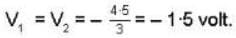*Multiple options can be correct
IIT JAM Physics MCQ Test 12 - Question 17

For the given circuit we have R1 = 6 kΩ, Rf = 24 kΩ, Vi = 1 V and load resistor of 6 kΩ. Then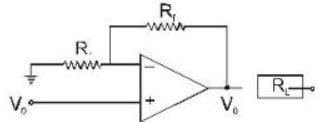Detailed Solution for IIT JAM Physics MCQ Test 12 - Question 17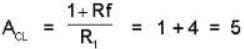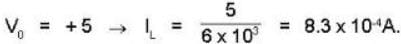*Multiple options can be correct
IIT JAM Physics MCQ Test 12 - Question 18

Fora differential amplifier in which the signal applied to inverting and non inverting term inals are respectively = - 0.45 m V and + 0.48 m V and A diff = 106 and CMRR = 80 dB.

Detailed Solution for IIT JAM Physics MCQ Test 12 - Question 18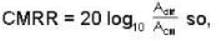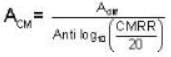So,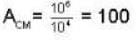The differential input is
Vdff = V2 - V1 = - 0.48 - (0.45) mV = -.03mV and common mode input is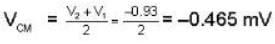Total output voltage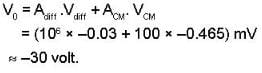*Multiple options can be correct
IIT JAM Physics MCQ Test 12 - Question 19

In a given n-type silicon material, the donar concentration is 1 atom per 2 * 108 silicon atoms. Assuming that the effective mass of the electron is equal to its true mass. Given that density of atoms in silicon = 5 * 1028 atoms/m3. Which of the following statement is correct.

Detailed Solution for IIT JAM Physics MCQ Test 12 - Question 19

The donor consecration will be calculated first. As there are 1 atoms per 2 x 10silicon atoms, there will be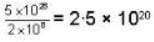donor atoms per met3. This density of doner
Nd = 2-5 x 1020 per m3
We know that Fermi level will coincides with the edge of conduction band if Nd = Nc, where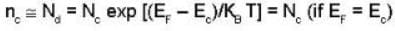with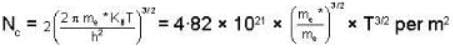But given that effective mass of the electron is equal to its true mass, i.e.,
me* = me
so that
NC = 4.82 x 1021 x T3/2
Putting it equal to Nd, we get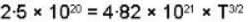or
T = 0.14 K

*Multiple options can be correct
IIT JAM Physics MCQ Test 12 - Question 20

For the circuit shown below:-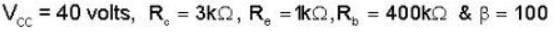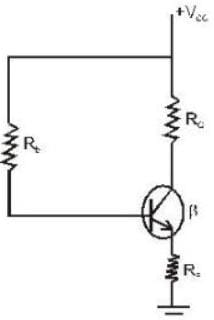Which of the following statement(s) is/are correct?

Detailed Solution for IIT JAM Physics MCQ Test 12 - Question 20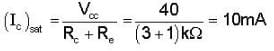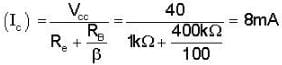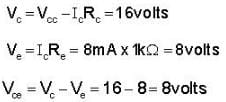*Answer can only contain numeric values
IIT JAM Physics MCQ Test 12 - Question 21

If a transistor has again of 20 dB, then the ratio of output to input power is_____.

Detailed Solution for IIT JAM Physics MCQ Test 12 - Question 21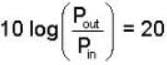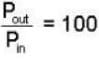*Answer can only contain numeric values
IIT JAM Physics MCQ Test 12 - Question 22

An CE transistor amplifier has a collector current of 1.0mA when its base current is 25μA at room temp. What is the value of its input resistance (in ohms)?

Detailed Solution for IIT JAM Physics MCQ Test 12 - Question 22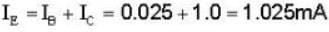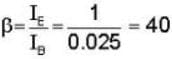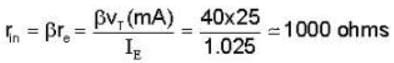*Answer can only contain numeric values
IIT JAM Physics MCQ Test 12 - Question 23

A transistor having α = 0.99 & VBE = 0.7V is used in the circuit shown figure. What is the value of collector current (in mA)?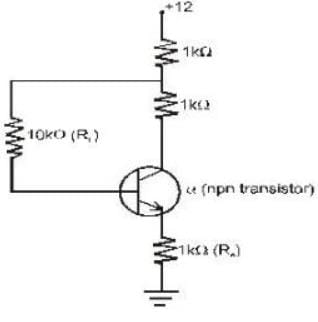Detailed Solution for IIT JAM Physics MCQ Test 12 - Question 23

Taking KVL for the base circuit;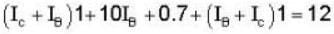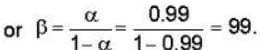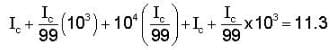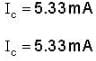*Answer can only contain numeric values
IIT JAM Physics MCQ Test 12 - Question 24

For the circuit,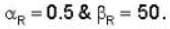If the saturation current is 10-16A . Find the value of base-emitter voltage (in V).

Detailed Solution for IIT JAM Physics MCQ Test 12 - Question 24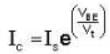Here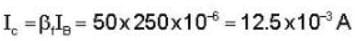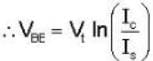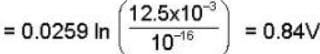*Answer can only contain numeric values
IIT JAM Physics MCQ Test 12 - Question 25

If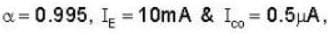then calculate the value of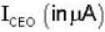?

Detailed Solution for IIT JAM Physics MCQ Test 12 - Question 25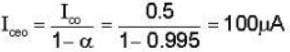*Answer can only contain numeric values
IIT JAM Physics MCQ Test 12 - Question 26

A String under a tension of 129.6 N produces 10 beats per sec when is vibrated along with a tuning frok. when tension in the string is increased to 160N, it sounds in unison with the same tuning frok. calculate the fundamental frequency of a tuning frok? (in Hz)

Detailed Solution for IIT JAM Physics MCQ Test 12 - Question 26

As here the tension in the wire is changed , so its fundamental frequency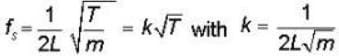Now with increase in tension,
(K√T) will increase & beats & decreasing to zero when
T = 160N (as union means frequencies are equal)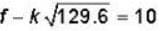...(i)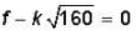...(ii)
Substituting the value of ‘k’ from second equation in first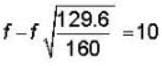f - 0.9 f = 10
f = 100Hz

*Answer can only contain numeric values
IIT JAM Physics MCQ Test 12 - Question 27

A column of air at 51oC & a tuning frok produce 4 beats per sec. when sounded togther. As the temperature of the air column is decreased, the number of beats sec tends to decrease & when the temperature is 16oc , the two produce 1 beat per sec find the frequency of the tuning frok (in Hz)

Detailed Solution for IIT JAM Physics MCQ Test 12 - Question 27

As for a given column of air f α v & V α √T
So ;  f = k√T
If the frequency of the frok is ‘f'
f - k √T = 4...(i)
OR
k√T - f = 4 ...(ii)
But as with decrease in temperature beats decrease, first choice is not permissible ;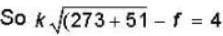i.e (f+4) = 18k ...(iii)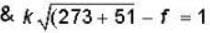i.e (f+1) = 17 k ...(iv)
So dividing eq(3) & (4) we get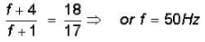*Answer can only contain numeric values
IIT JAM Physics MCQ Test 12 - Question 28

Calculate the thickness of a soap bubble film (refractive index μ =1.3) that will result in constructive interference in the reflected light if the film is illuminated with light whose wavelength in free space is 5000 A0 (Thickness (+) is in Ao)?

Detailed Solution for IIT JAM Physics MCQ Test 12 - Question 28

The condition for constructive interference in the reflected light or fro the brightness of soap bubble film is:-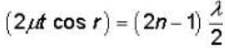When, n = 0 ,1 ,2 ,3 ..............
If the light is incident normally on the film, then r = 0
& cos r = 1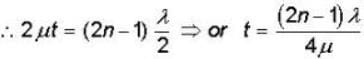putting the given values , we get:-
for least thickness of the film (n=1)
∴ least thickness of the film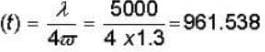t = 961.53 A0

*Answer can only contain numeric values
IIT JAM Physics MCQ Test 12 - Question 29

In the given circuit Vcc = 8V and β = 80 for the n-p-n transistor.The collector voltage V(in volts) is_____.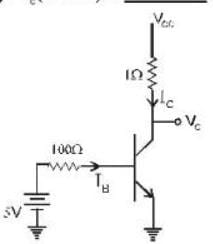Detailed Solution for IIT JAM Physics MCQ Test 12 - Question 29

Applying KVL
5 — 100IB - 0.7 = 0
4.3/100 = IB
I= 43mA
Ic = 80X 43mA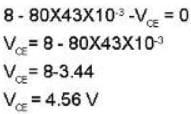*Answer can only contain numeric values
IIT JAM Physics MCQ Test 12 - Question 30

The maximum value of RL so that zener diode is 'ON ' is______(in kΩ).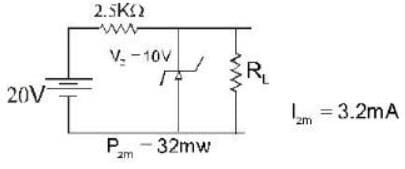Detailed Solution for IIT JAM Physics MCQ Test 12 - Question 30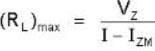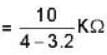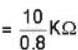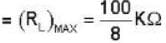= 12.5KΩ

## Mock Test Series for IIT JAM Physics

1 docs|21 tests
 Use Code STAYHOME200 and get INR 200 additional OFF Use Coupon Code
Information about IIT JAM Physics MCQ Test 12 Page
In this test you can find the Exam questions for IIT JAM Physics MCQ Test 12 solved & explained in the simplest way possible. Besides giving Questions and answers for IIT JAM Physics MCQ Test 12, EduRev gives you an ample number of Online tests for practice

1 docs|21 tests